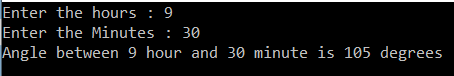# How to find the angle between hour and minute hands of a clock at any given time in C#

## How to find the angle between the hour and minute hands of a clock at any given time in C#

In this article, I am going to discuss how to find the angle between the hour and minute hands of a clock at any given time in C# with an example. Please read our previous article where we discussed How to Perform Right Circular Rotation of an Array in C# with an example.

##### Program Description:

Here, the user will input the hour and minute. Then we need to calculate the angle between the given hours and minute. For example, if the user input as 9hour 30 minutes, then the angle between the hour hand and minute hand should be 105 degrees. If the input is 13 hour 30 minutes then the output should be 135 degrees

##### Finding the angle between the hour and minute hands of a clock at any given time:

The logic that we need to implement is to find the difference in the angle of an hour and minute hand from the position of 12 O Clock when the angle between them is zero. Each hour on the clock represents an angle of 30 degrees (360 divided by 12). Similarly, each minute on the clock will represent an angle of 6 degrees (360 divided by 60) and the angle for an hour will increase as the minutes for that hour increases.

```using System;
namespace LogicalPrograms
{
class Program
{
static void Main(string[] args)
{
Console.Write("Enter the hours : ");
Console.Write("Enter the Minutes : ");

double hourInDegrees = (hours * 30) + (minutes * 30.0 / 60);
double minuteInDegrees = minutes * 6;

double diff = Math.Abs(hourInDegrees - minuteInDegrees);

if (diff > 180)
{
diff = 360 - diff;
}

Console.WriteLine(\$"Angle between {hours} hour and {minutes} minute is {diff} degrees");